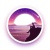0+0+0+0+0+0+0+0+0+0+0+0+0+0+0+0+0+0+0+0+0+0+0+0+0+0+0+0+1+0+0+0+0+0+0+0+0+1+0+0+0+0+0+0+0+
Top